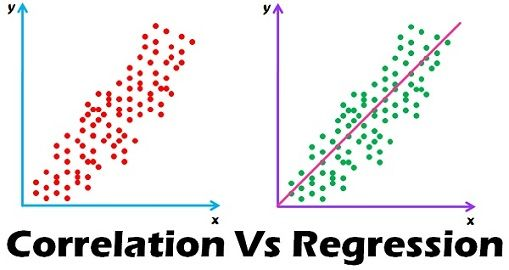#Correlation and Regression are the two analysis based on multivariate distribution. A multivariate distribution is described as a distribution of multiple variables. Correlation is described as the analysis which lets us know the association or the absence of the relationship between two variables ‘x’ and ‘y’. On the other end, Regression analysis, predicts the value of the dependent variable based on the known value of the independent variable, assuming that average mathematical relationship between two or more variables.

The difference between correlation and regression is one of the commonly asked questions in interviews. Moreover, many people suffer ambiguity in understanding these two. So, take a full read of this article to have a clear understanding on these two.

### Comparison Chart

Basis for Comparison Correlation Regression
Meaning Correlation is a statistical measure which determines co-relationship or association of two variables. Regression describes how an independent variable is numerically related to the dependent variable.
Usage To represent linear relationship between two variables. To fit a best line and estimate one variable on the basis of another variable.
Dependent and Independent variables No difference Both variables are different.
Indicates Correlation coefficient indicates the extent to which two variables move together. Regression indicates the impact of a unit change in the known variable (x) on the estimated variable (y).
Objective To find a numerical value expressing the relationship between variables. To estimate values of random variable on the basis of the values of fixed variable.

Views: 128071

Comment

Join Data Science CentralComment by Dan Butorovich on March 28, 2018 at 11:18am

Actually Alok, Asim is correct in his article. The correlation coefficient of your x and y is .975, I got the same result whether calculated by hand using the Pearson formula or calculated using R's cor(). Just because each y is a multiple or square of its corresponding x doesn't mean that it isn't estimable by a linear equation, or that they don't co-vary. In the case where you have truly nonlinear data, you can use other non-Pearson correlations such as Kendall's Tau, or Spearman's equations. Correlation is also about covariance, how much the two things vary together. As x changes, y changes and they do so together within the limits of the observation. Regression demands linearity, correlation less so as long as the two variables vary together to some measurable degree.Comment by Asim Jana on March 27, 2018 at 5:08pm

Hi Alok,

Very effective comment. See my below comments.

Two variables are said to be "correlated" or "associated" if knowing scores for one of them helps to predict scores for the other.  Capacity to predict is measured by a correlation coefficient that can indicate some amount of relationship, no relationship, or some amount of inverse relationship between the variables.Comment by Alok Kumar on March 21, 2018 at 9:38pm

"Correlation coefficient indicates the extent to which two variables move together." - not really.

Illustration - x (1,2,3,4,5,6,7,8, 9) and y (1,4,9,16,25,36,49,64,81) -  x and y here move together. But what is the correlation coefficient? Even a Statistics Graduate passed out from the best of the colleges tend to say there is perfect correlation between the two. Actually not !  There is no correlation between the 2 variables. Don't you believe me? Calculate the Corr Coeff and what you will get may surprise you.

Correlation Coefficient shows the extent to which they are "linearly" related ie the relationship between the two variables can be in expressed in the form of a straight line. Correlation is just a step on the way to regression.